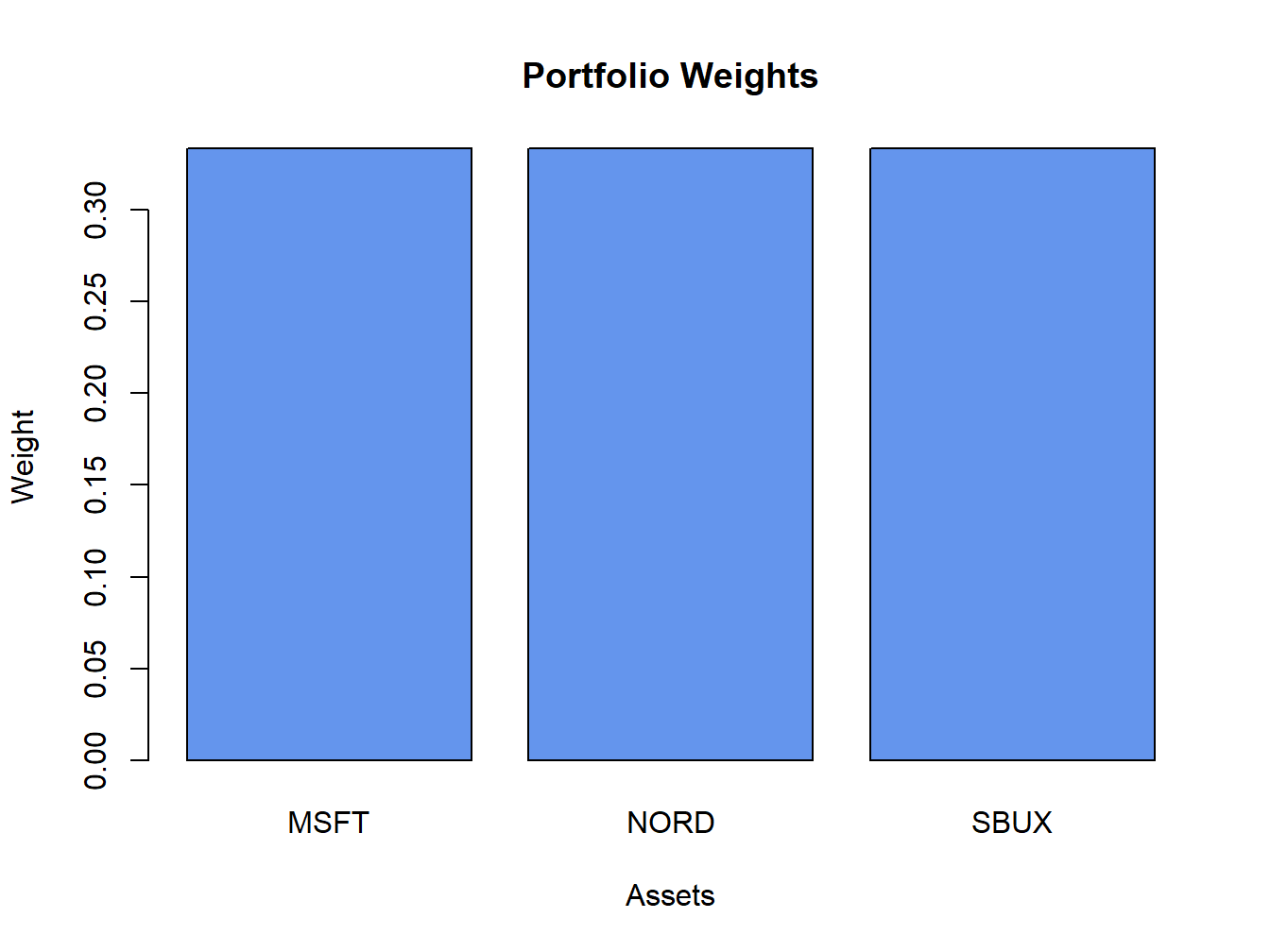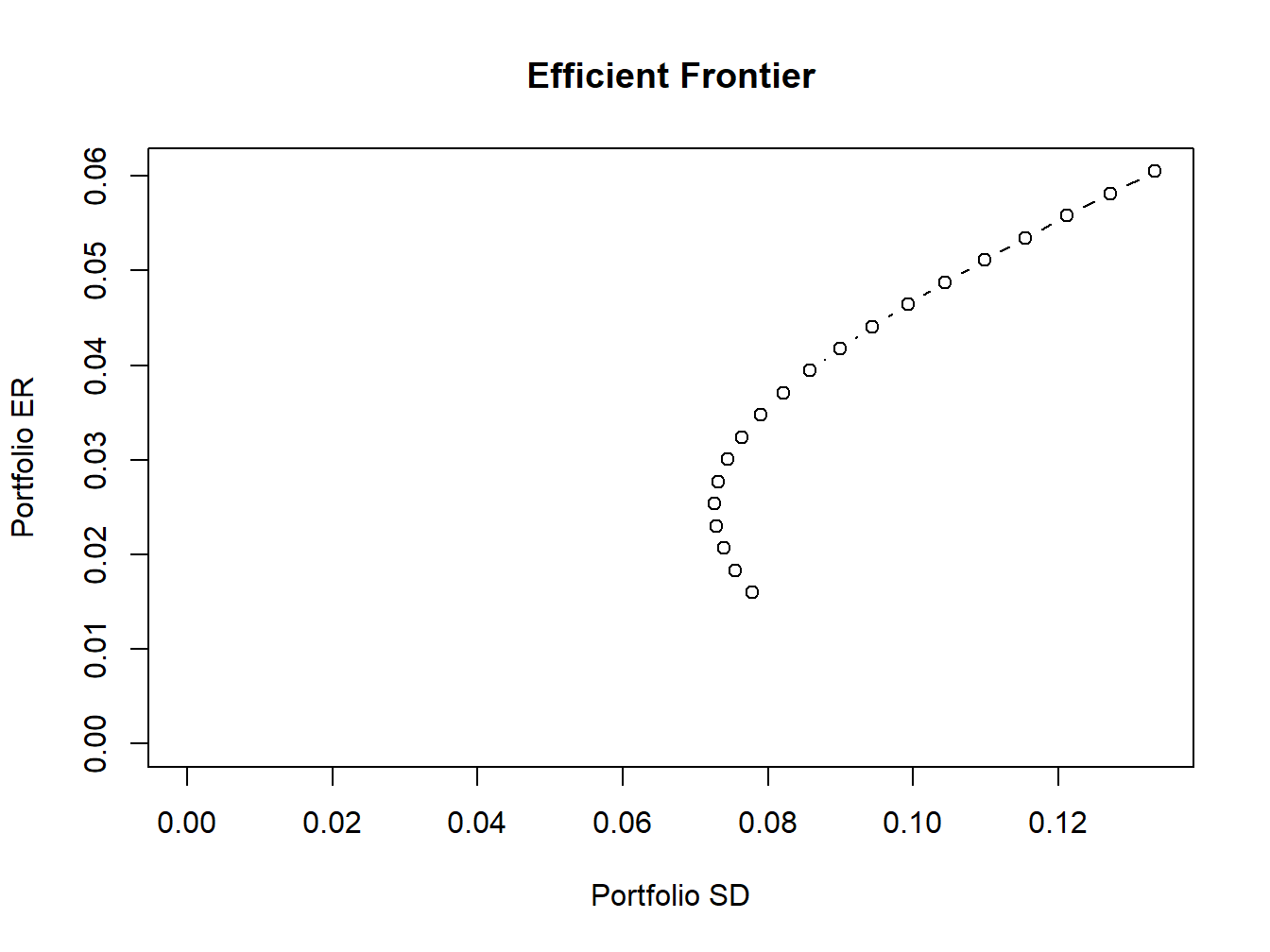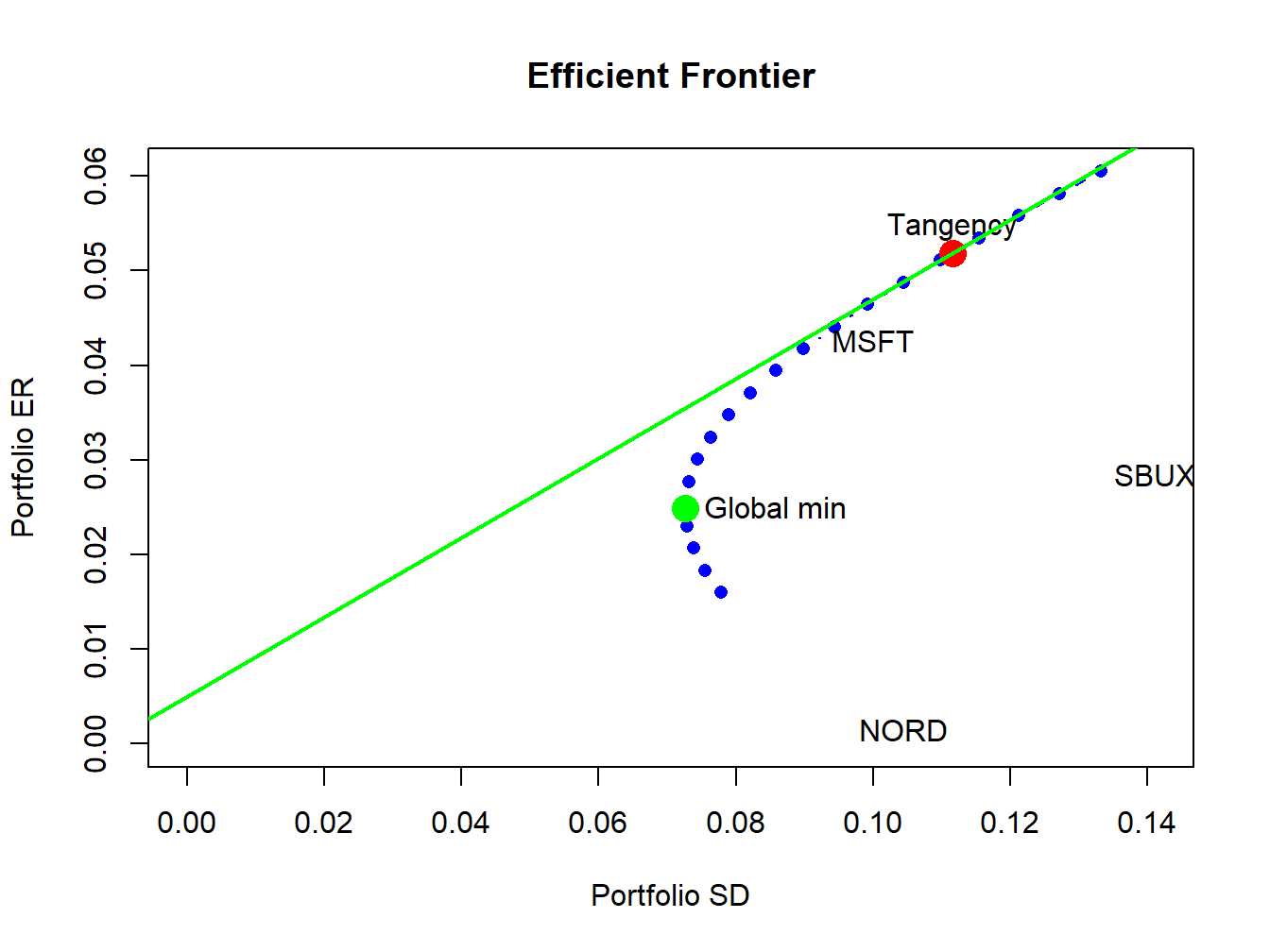## 12.7 Portfolio Analysis Functions in R

The package IntroCompFinR contains a few R functions for computing Markowitz mean-variance efficient portfolios allowing for short sales using matrix algebra computations. These functions allow for the easy computation of the global minimum variance portfolio, an efficient portfolio with a given target expected return, the tangency portfolio, and the efficient frontier. These functions are summarized in Table 12.2.

Table 12.2: IntroCompFinR functions for computing mean-variance efficient portfolios
Function Description
getPortfolio create portfolio object
globalMin.portfolio compute global minimum variance portfolio
efficient.portfolio compute minimum variance portfolio subject to target return
tangency.portfolio compute tangency portfolio
efficient.frontier compute efficient frontier of risky assets

The following examples illustrate the use of the functions in Table 12.2 using the example data in Table 12.1:

mu.vec
##   MSFT   NORD   SBUX
## 0.0427 0.0015 0.0285
sigma.mat
##        MSFT   NORD   SBUX
## MSFT 0.0100 0.0018 0.0011
## NORD 0.0018 0.0109 0.0026
## SBUX 0.0011 0.0026 0.0199
r.f
##  0.005

To specify a portfolio object, you need an expected return vector and covariance matrix for the assets under consideration as well as a vector of portfolio weights. To create an equally weighted portfolio use:

library(IntroCompFinR)
ew = rep(1,3)/3
equalWeight.portfolio = getPortfolio(er=mu.vec,cov.mat=sigma.mat,weights=ew)
class(equalWeight.portfolio)
##  "portfolio"

Portfolio objects have the following components:

names(equalWeight.portfolio)
##  "call"    "er"      "sd"      "weights"

There are print(), summary() and plot() methods for portfolio objects. The print() method gives:

equalWeight.portfolio
## Call:
## getPortfolio(er = mu.vec, cov.mat = sigma.mat, weights = ew)
##
## Portfolio expected return:     0.0242
## Portfolio standard deviation:  0.0759
## Portfolio weights:
##  MSFT  NORD  SBUX
## 0.333 0.333 0.333

The plot() method shows a bar chart of the portfolio weights:

plot(equalWeight.portfolio, col="cornflowerblue")Figure 12.11: Plot method for objects of class “portfolio”

The resulting plot is shown in Figure 12.11. The global minimum variance portfolio (allowing for short sales) $$\mathbf{m}$$ solves the optimization problem (12.2) and is computed using (12.6). To compute this portfolio use the function globalMin.portfolio():

gmin.port = globalMin.portfolio(mu.vec, sigma.mat)
class(gmin.port)
##  "portfolio"
gmin.port
## Call:
## globalMin.portfolio(er = mu.vec, cov.mat = sigma.mat)
##
## Portfolio expected return:     0.0249
## Portfolio standard deviation:  0.0727
## Portfolio weights:
##  MSFT  NORD  SBUX
## 0.441 0.366 0.193

A mean-variance efficient portfolio $$\mathbf{x}$$ that achieves the target expected return $$\mu_{0}$$ solves the optimization problem (12.10) and is computed using (12.14). To compute this portfolio for the target expected return $$\mu_{0}=E[R_{\textrm{msft}}]=0.0427$$ use the efficient.portfolio() function:

target.return = mu.vec
e.port.msft = efficient.portfolio(mu.vec, sigma.mat, target.return)
class(e.port.msft)
##  "portfolio"
e.port.msft
## Call:
## efficient.portfolio(er = mu.vec, cov.mat = sigma.mat, target.return = target.return)
##
## Portfolio expected return:     0.0427
## Portfolio standard deviation:  0.0917
## Portfolio weights:
##    MSFT    NORD    SBUX
##  0.8275 -0.0907  0.2633

The tangency portfolio $$\mathbf{t}$$ is the portfolio of risky assets with the highest Sharpe’s slope and has solutions given by (12.26). To compute this portfolio with $$r_{f}=0.005$$ use the tangency.portfolio() function:

tan.port = tangency.portfolio(mu.vec, sigma.mat, r.f)
class(tan.port)
##  "portfolio"
tan.port
## Call:
## tangency.portfolio(er = mu.vec, cov.mat = sigma.mat, risk.free = r.f)
##
## Portfolio expected return:     0.0519
## Portfolio standard deviation:  0.112
## Portfolio weights:
##   MSFT   NORD   SBUX
##  1.027 -0.326  0.299

The the set of efficient portfolios of risky assets can be computed as a convex combination of any two efficient portfolios. It is convenient to use the global minimum variance portfolio as one portfolio and an efficient portfolio with target expected return equal to the maximum expected return of the assets under consideration as the other portfolio. Call these portfolios $$\mathbf{m}$$ and $$\mathbf{x}$$, respectively. For any number $$\alpha$$, another efficient portfolio can be computed as: $\mathbf{z}=\alpha\mathbf{x}+(1-\alpha)\mathbf{m}$ The function efficient.frontier() constructs the set of efficient portfolios using this method for a collection of $$\alpha$$ values on an equally spaced grid between $$\alpha_{min}$$ and $$\alpha_{max}$$. For example, to compute 20 efficient portfolios for values of $$\alpha$$ between -2 and 1.5 use:

ef = efficient.frontier(mu.vec, sigma.mat, alpha.min=-0.5,
alpha.max=2, nport=20)
attributes(ef)
## $names ##  "call" "er" "sd" "weights" ## ##$class
##  "Markowitz"
ef
## Call:
## efficient.frontier(er = mu.vec, cov.mat = sigma.mat, nport = 20,
##     alpha.min = -0.5, alpha.max = 2)
##
## Frontier portfolios' expected returns and standard deviations
##    port 1 port 2 port 3 port 4 port 5 port 6 port 7 port 8 port 9 port 10
## ER 0.0160 0.0183 0.0207 0.0230 0.0254 0.0277 0.0300 0.0324 0.0347  0.0371
## SD 0.0779 0.0755 0.0739 0.0729 0.0727 0.0732 0.0745 0.0764 0.0790  0.0821
##    port 11 port 12 port 13 port 14 port 15 port 16 port 17 port 18 port 19
## ER  0.0394  0.0418  0.0441  0.0464  0.0488  0.0511  0.0535  0.0558  0.0582
## SD  0.0858  0.0899  0.0944  0.0993  0.1044  0.1098  0.1154  0.1212  0.1272
##    port 20
## ER  0.0605
## SD  0.1333

Use the summary() method to show the weights of these portfolios. Use the plot() method to create a simple plot the efficient frontier:

plot(ef)Figure 12.12: Plot method for objects of class “Markowitz”

The resulting plot is shown in Figure 12.12. To create a more elaborate plot of the efficient frontier showing the original assets and the tangency portfolio use:

plot(ef, plot.assets=T, col="blue", pch=16)
points(gmin.port$sd, gmin.port$er, col="green", pch=16, cex=2)
text(gmin.port$sd, gmin.port$er, labels = "Global min", pos = 4)
points(tan.port$sd, tan.port$er, col="red", pch=16, cex=2)
text(tan.port$sd, tan.port$er, labels = "Tangency", pos = 3)
sr.tan = (tan.port$er - r.f)/tan.port$sd
abline(a=r.f, b=sr.tan, col="green", lwd=2)Figure 12.13: Efficient frontier plot.

The resulting plot is shown in Figure 12.13.